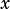What value ofsatisfies the equation above?Ilikedots1 says:

If A=x-3 then 0=a^2-6a or 0=a(a-6). A=0 or 6. Plug 6 in for A and get x=9. Plug 0 in for A and get x=3. I believe the answers should be 6 or 9.Ilikedots1 says:

I meant x=3 or 9 lol.Kubbs says:

You can actually graph the function above and the only solution is x=6. You seem to be mixing up some things.

Let us say, like you did, that a=(x-3)
Therefore, (x-3)^2 – 6(x-3) + 9 would be translated into a^2 – 6a +9. NOT a^2 – 6a like you suggested. (You left out the 9)

Now lets continue to solve this:
0 = a^2 – 6a + 9
0 = (a-3)^2

There is only one solution here to “a”. a=3
so…
a=3 substitute x-3 in for a
(x-3)=3 add three to both sides
x=6infoanalysis says:

I did it the non sub way it came out just as fast

( x-3)^2 = x^2 -6x +9
x^2 -6x +9 -6 (x-3) +9
X^2-6x+9-6x-+18+9
X^2-12x+36
(X-6)^2

x=6aarhin10 says:

I did it the same way as you did and got x = 6 as well 👍aarhin10 says:

Nvm mine was slightly different lolaarhin10 says:

I did it this way and got the same answer quickly.

0 = (x-3)^2 – 6(x-3) + 9
0 = (x-3)(x-3) -(6x-18) + 9
0 = x^2 -6x + 9 – 6x + 18 +9
0 = x^2 – 12x + 36
0 = (x-6)(x-6)
x = 6